# 324 Project 3 released Due in two weeks

• Slides: 32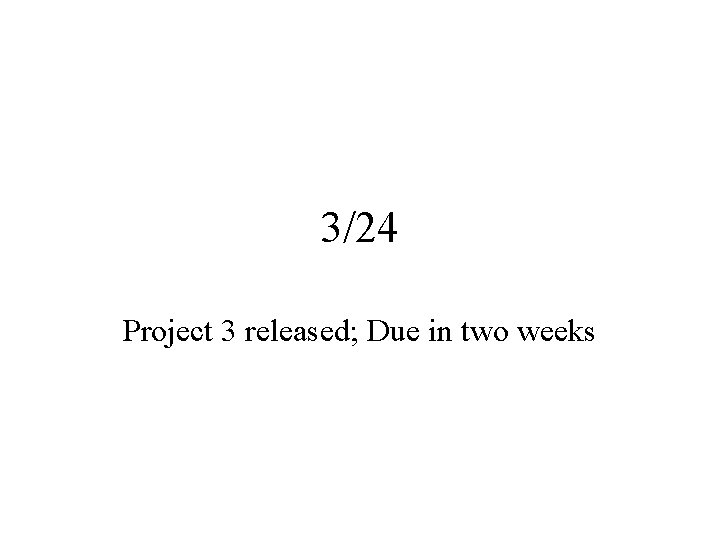3/24 Project 3 released; Due in two weeks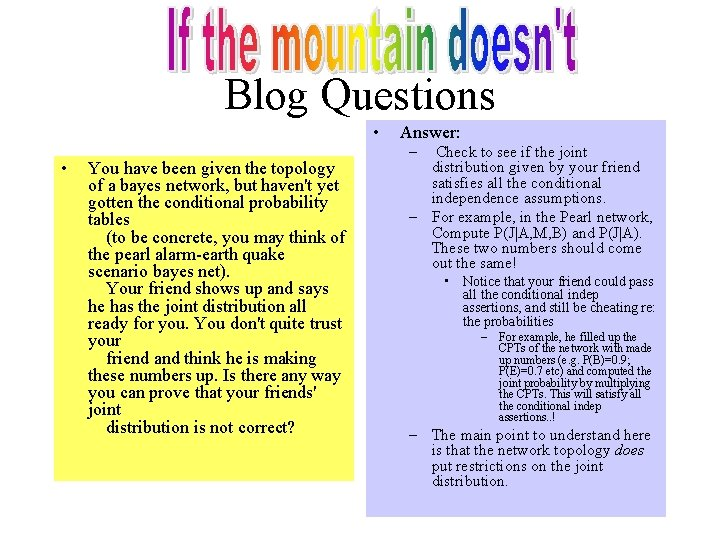Blog Questions • • You have been given the topology of a bayes network, but haven't yet gotten the conditional probability tables (to be concrete, you may think of the pearl alarm-earth quake scenario bayes net). Your friend shows up and says he has the joint distribution all ready for you. You don't quite trust your friend and think he is making these numbers up. Is there any way you can prove that your friends' joint distribution is not correct? Answer: – Check to see if the joint distribution given by your friend satisfies all the conditional independence assumptions. – For example, in the Pearl network, Compute P(J|A, M, B) and P(J|A). These two numbers should come out the same! • Notice that your friend could pass all the conditional indep assertions, and still be cheating re: the probabilities – For example, he filled up the CPTs of the network with made up numbers (e. g. P(B)=0. 9; P(E)=0. 7 etc) and computed the joint probability by multiplying the CPTs. This will satisfy all the conditional indep assertions. . ! – The main point to understand here is that the network topology does put restrictions on the joint distribution.Blog Questions (2) • • Continuing bad friends, in the question above, suppose a second friend comes along and says that he can give you the conditional probabilities that you want to complete the specification of your bayes net. You ask him a CPT entry, and pat comes a response--some number between 0 and 1. This friend is well meaning, but you are worried that the numbers he is giving may lead to some sort of inconsistent joint probability distribution. Is your worry justified ( i. e. , can your friend give you numbers that can lead to an inconsistency? ) (To understand "inconsistency", consider someone who insists on giving you P(A), P(B), P(A&B) as well as P(AVB) and they wind up not satisfying the P(AVB)= P(A)+P(B) -P(A&B) [or alternately, they insist on giving you P(A|B), P(B|A), P(A) and P(B), and the four numbers dont satisfy the bayes rule] • Answer: No—as long as we only ask the friend to fill up the CPTs in the bayes network, there is no way the numbers won’t makeup a consistent joint probability distribution – This should be seen as a feature. . Personal Probabilities – John may be an optimist and believe that P(burglary)=0. 01 and Tom may be a pessimist and believe that P(burglary)=0. 99 – Bayesians consider both John and Tom to be fine (they don’t insist on an objective frequentist interpretation for probabilites) – However, Bayesians do think that John and Tom should act consistently with their own beliefs • For example, it makes no sense for John to go about installing tons of burglar alarms given his belief, just as it makes no sense for Tom to put all his valuables on his lawn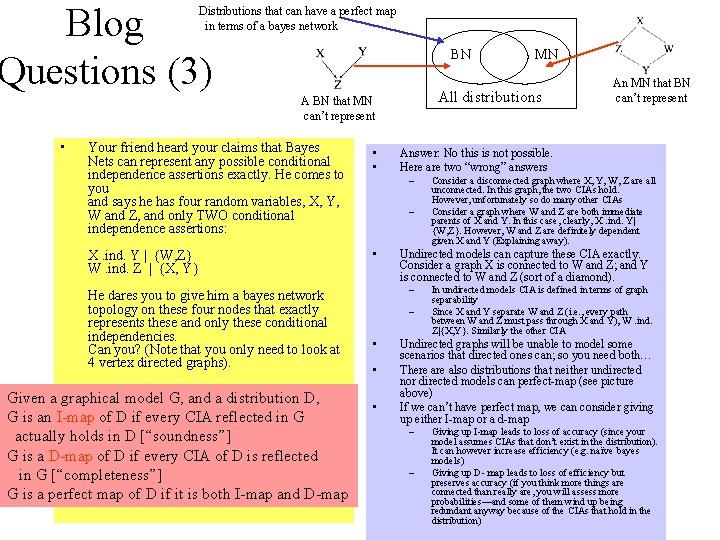Blog Questions (3) Distributions that can have a perfect map in terms of a bayes network BN All distributions A BN that MN can’t represent • Your friend heard your claims that Bayes Nets can represent any possible conditional independence assertions exactly. He comes to you and says he has four random variables, X, Y, W and Z, and only TWO conditional independence assertions: • • X. ind. Y | {W, Z} W. ind. Z | {X, Y} • He dares you to give him a bayes network topology on these four nodes that exactly represents these and only these conditional independencies. Can you? (Note that you only need to look at 4 vertex directed graphs). Given a graphical model G, and a distribution D, G is an I-map of D if every CIA reflected in G actually holds in D [“soundness”] G is a D-map of D if every CIA of D is reflected in G [“completeness”] G is a perfect map of D if it is both I-map and D-map – – • Consider a disconnected graph where X, Y, W, Z are all unconnected. In this graph, the two CIAs hold. However, unfortunately so do many other CIAs Consider a graph where W and Z are both immediate parents of X and Y. In this case, clearly, X. ind. Y| {W, Z}. However, W and Z are definitely dependent given X and Y (Explaining away). Undirected models can capture these CIA exactly. Consider a graph X is connected to W and Z; and Y is connected to W and Z (sort of a diamond). – • An MN that BN can’t represent Answer: No this is not possible. Here are two “wrong” answers – • MN In undirected models CIA is defined in terms of graph separability Since X and Y separate W and Z (i. e. , every path between W and Z must pass through X and Y), W. ind. Z|{X, Y}. Similarly the other CIA Undirected graphs will be unable to model some scenarios that directed ones can; so you need both… There also distributions that neither undirected nor directed models can perfect-map (see picture above) If we can’t have perfect map, we can consider giving up either I-map or a d-map – – Giving up I-map leads to loss of accuracy (since your model assumes CIAs that don’t exist in the distribution). It can however increase efficiency (e. g. naïve bayes models) Giving up D- map leads to loss of efficiency but preserves accuracy (if you think more things are connected than really are, you will assess more probabilities—and some of them wind up being redundant anyway because of the CIAs that hold in the distribution)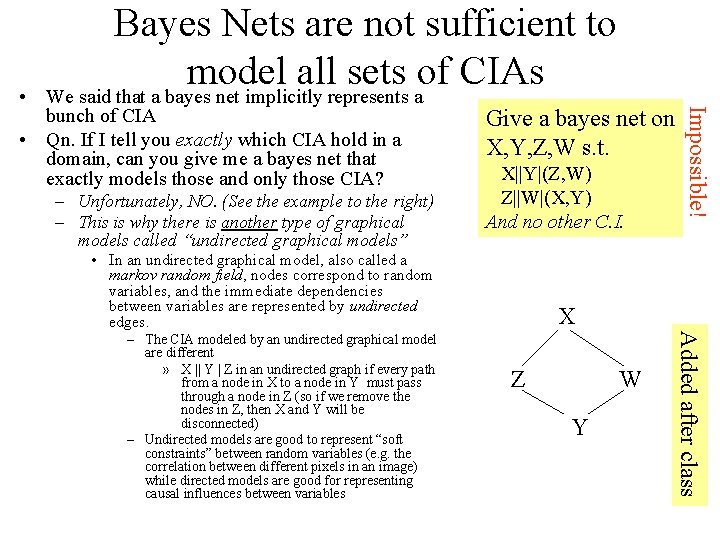bunch of CIA • Qn. If I tell you exactly which CIA hold in a domain, can you give me a bayes net that exactly models those and only those CIA? – Unfortunately, NO. (See the example to the right) – This is why there is another type of graphical models called “undirected graphical models” Give a bayes net on X, Y, Z, W s. t. X||Y|(Z, W) Z||W|(X, Y) And no other C. I. • In an undirected graphical model, also called a markov random field, nodes correspond to random variables, and the immediate dependencies between variables are represented by undirected edges. X Z W Y Added after class – The CIA modeled by an undirected graphical model are different » X || Y | Z in an undirected graph if every path from a node in X to a node in Y must pass through a node in Z (so if we remove the nodes in Z, then X and Y will be disconnected) – Undirected models are good to represent “soft constraints” between random variables (e. g. the correlation between different pixels in an image) while directed models are good for representing causal influences between variables Impossible! • Bayes Nets are not sufficient to model all sets of CIAs We said that a bayes net implicitly represents aFactorization as the basis for Graphical Models • Both Bayes Nets and Markov Nets can be thought of representing joint distributions that have a particular way of being factorized – Analogy: Think of an integer that can be factorized in a certain way • Bayes nets The factors are CPTs (for each node given its immediate parents). Joint distribution is the product of CPTs. – Analogy: Think of an integer that can be factorized into a product of 4 unique prime numbers. Added after class • Markov nets The factors are potential functions (for cliques of nodes in the net, we give “numbers” roughly representing the weight for each of their joint configurations. ). They have no probabilistic interpretation. The joint is the normalized product of these potential functions.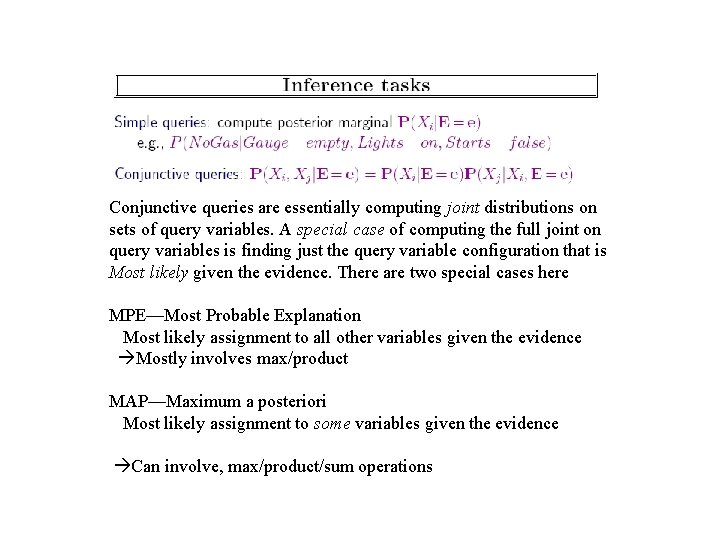Conjunctive queries are essentially computing joint distributions on sets of query variables. A special case of computing the full joint on query variables is finding just the query variable configuration that is Most likely given the evidence. There are two special cases here MPE—Most Probable Explanation Most likely assignment to all other variables given the evidence Mostly involves max/product MAP—Maximum a posteriori Most likely assignment to some variables given the evidence Can involve, max/product/sum operations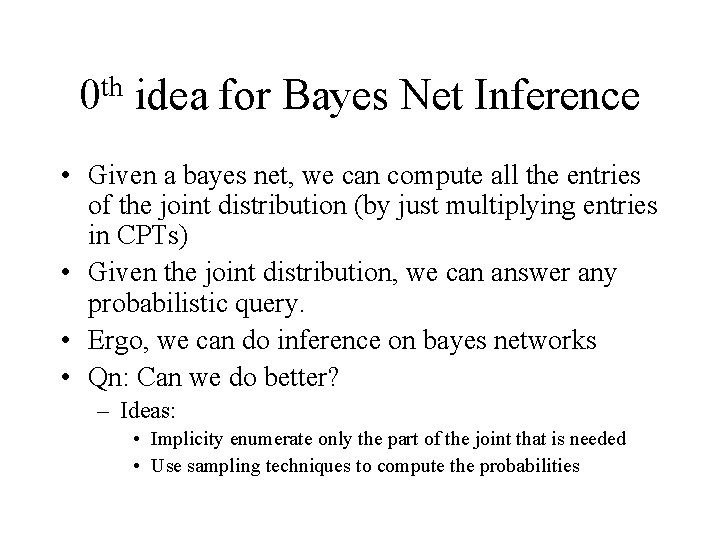th 0 idea for Bayes Net Inference • Given a bayes net, we can compute all the entries of the joint distribution (by just multiplying entries in CPTs) • Given the joint distribution, we can answer any probabilistic query. • Ergo, we can do inference on bayes networks • Qn: Can we do better? – Ideas: • Implicity enumerate only the part of the joint that is needed • Use sampling techniques to compute the probabilitiesNetwork Topology & Complexity of Inference Sprinklers Rain Wetgrass Can be converted to singly-connected (by merging nodes) Cloudy Singly Connected Networks (poly-trees – At most one path between any pair of nodes) Inference is polynomial Wetgrass Sprinklers+Rain (takes 4 values 2 x 2) The “size” of the merged network can be Exponentially larger (so polynomial inference on that network isn’t exactly god’s gift Cloudy Multiply connected Inference NP-hard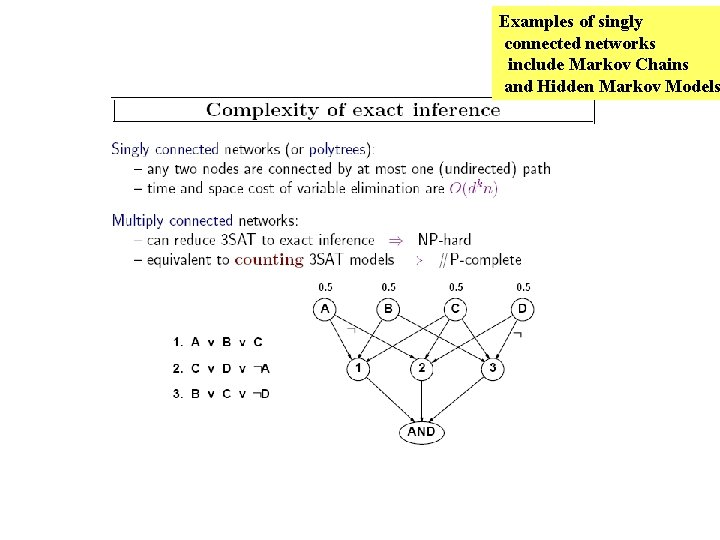Examples of singly connected networks include Markov Chains and Hidden Markov Models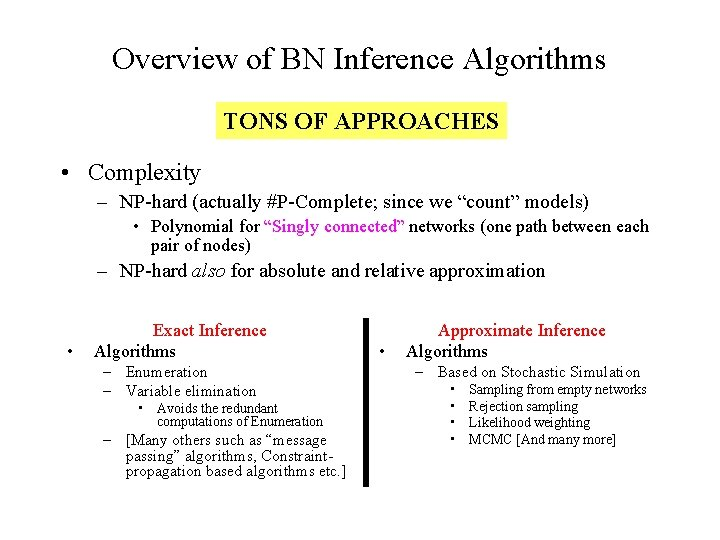Overview of BN Inference Algorithms TONS OF APPROACHES • Complexity – NP-hard (actually #P-Complete; since we “count” models) • Polynomial for “Singly connected” networks (one path between each pair of nodes) – NP-hard also for absolute and relative approximation • Exact Inference Algorithms – Enumeration – Variable elimination • Avoids the redundant computations of Enumeration – [Many others such as “message passing” algorithms, Constraintpropagation based algorithms etc. ] • Approximate Inference Algorithms – Based on Stochastic Simulation • • Sampling from empty networks Rejection sampling Likelihood weighting MCMC [And many more]3/26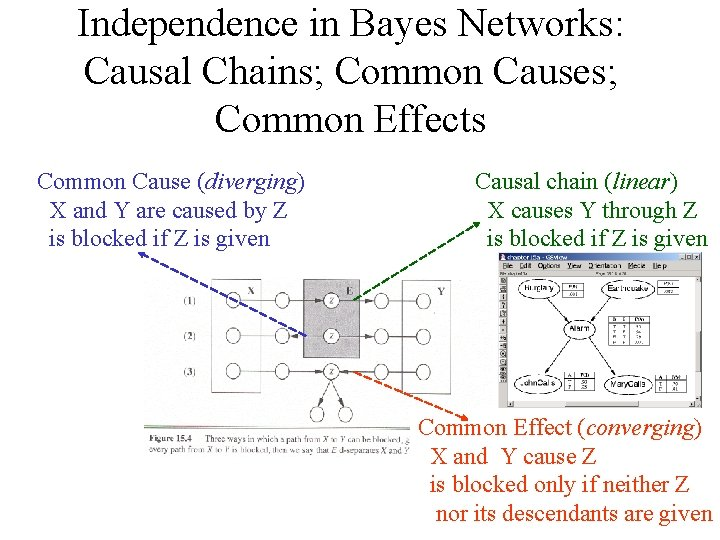Independence in Bayes Networks: Causal Chains; Common Causes; Common Effects Common Cause (diverging) X and Y are caused by Z is blocked if Z is given Causal chain (linear) X causes Y through Z is blocked if Z is given Common Effect (converging) X and Y cause Z is blocked only if neither Z nor its descendants are given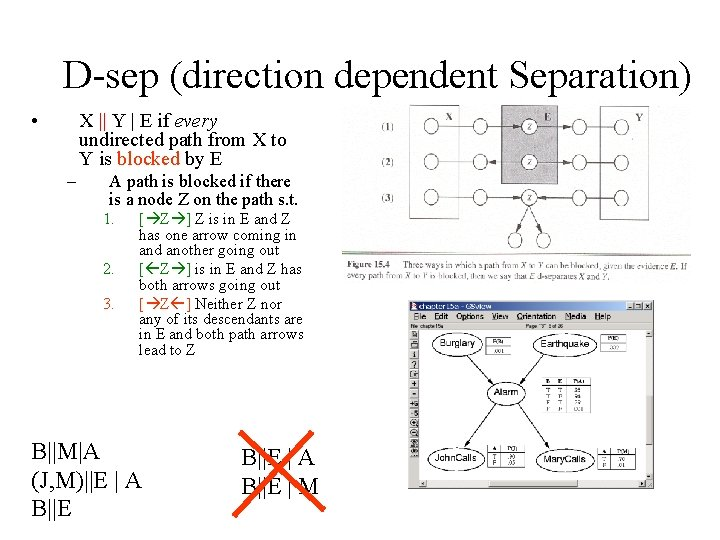D-sep (direction dependent Separation) • X || Y | E if every undirected path from X to Y is blocked by E – A path is blocked if there is a node Z on the path s. t. 1. 2. 3. [ Z ] Z is in E and Z has one arrow coming in and another going out [ Z ] is in E and Z has both arrows going out [ Z ] Neither Z nor any of its descendants are in E and both path arrows lead to Z B||M|A (J, M)||E | A B||E | M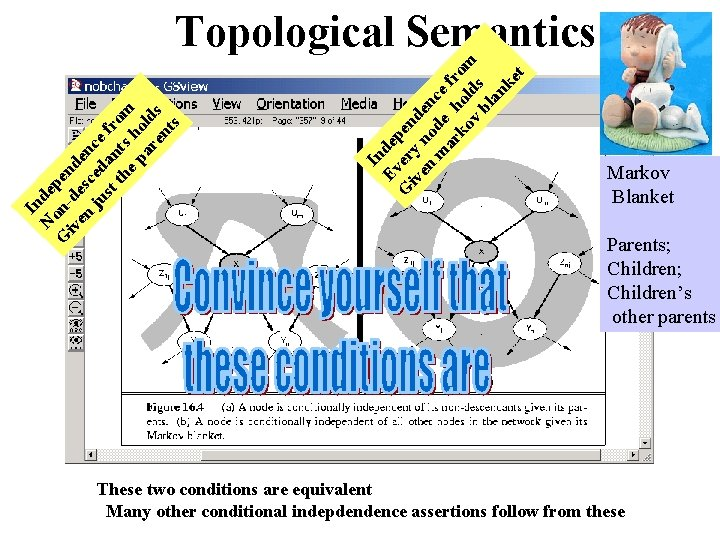In Ev dep G er en iv y en no den m de ce ar h fr ko ol om v ds bl an ke t In N dep G on- en iv de d en sc en ju ed ce st an fr th ts om ep h ar old en s ts Topological Semantics Markov Blanket Parents; Children’s other parents These two conditions are equivalent Many other conditional indepdendence assertions follow from theseIf the expression tree is evaluated in a depth first fashion, then the space requirement is linear. .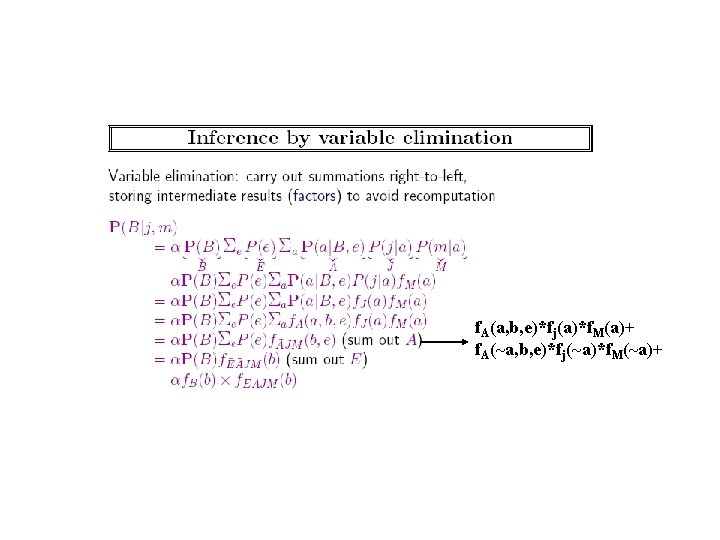f. A(a, b, e)*fj(a)*f. M(a)+ f. A(~a, b, e)*fj(~a)*f. M(~a)+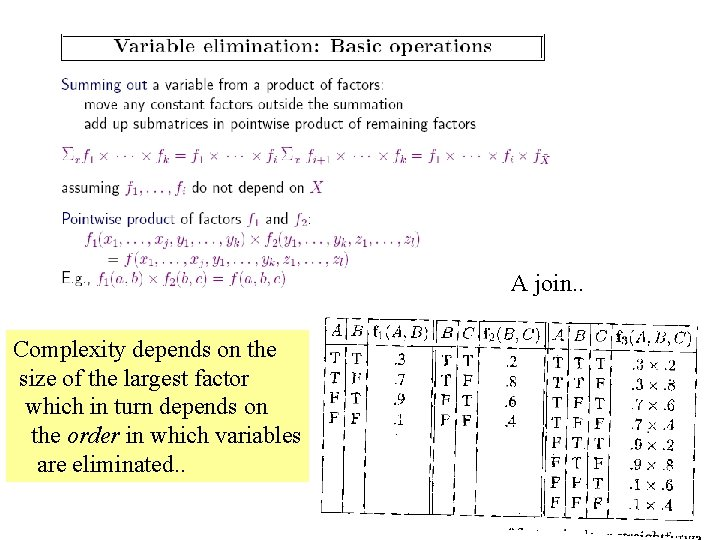A join. . Complexity depends on the size of the largest factor which in turn depends on the order in which variables are eliminated. .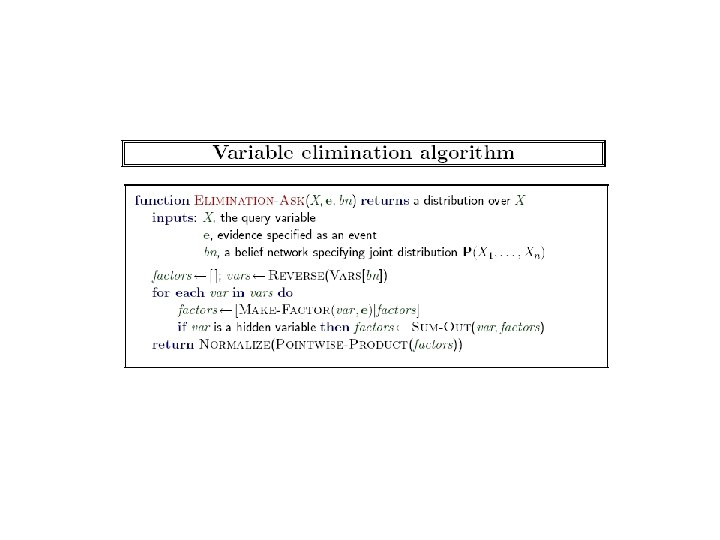*Read Worked Out Example of Variable Elimination in the Lecture Notes*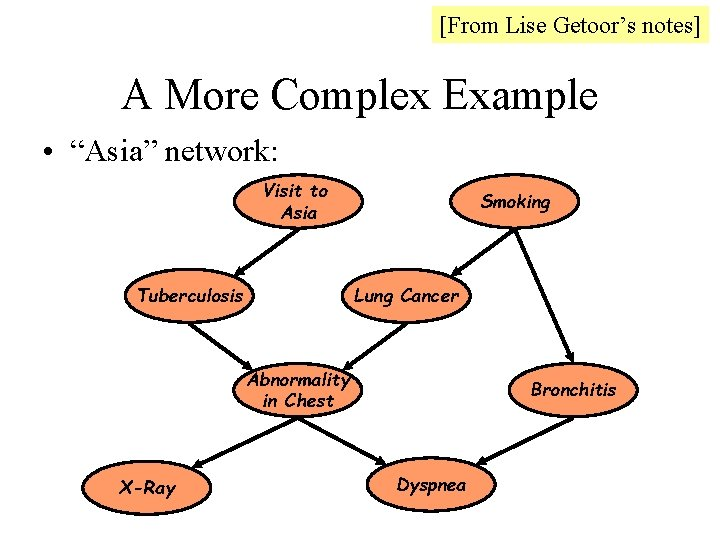[From Lise Getoor’s notes] A More Complex Example • “Asia” network: Visit to Asia Tuberculosis Smoking Lung Cancer Abnormality in Chest X-Ray Bronchitis Dyspnea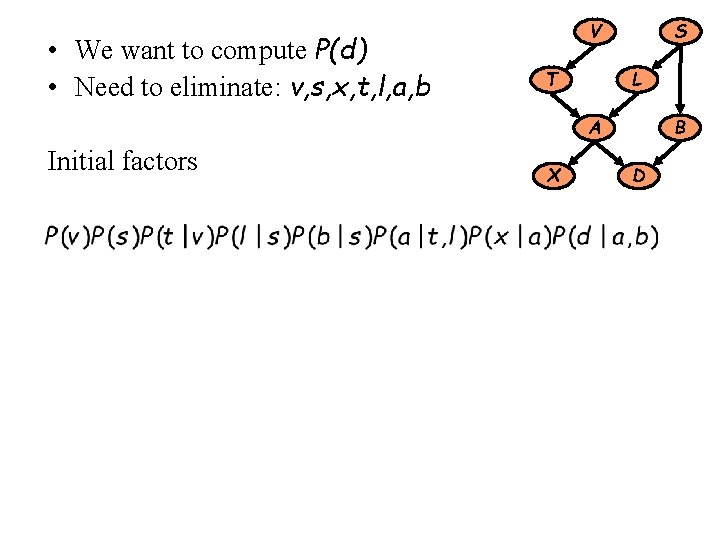• We want to compute P(d) • Need to eliminate: v, s, x, t, l, a, b S V L T B A Initial factors X D• We want to compute P(d) • Need to eliminate: v, s, x, t, l, a, b S V L T B A Initial factors X D Eliminate: v Compute: Note: fv(t) = P(t) In general, result of elimination is not necessarily a probability term• We want to compute P(d) • Need to eliminate: s, x, t, l, a, b S V L T B A • Initial factors X D Eliminate: s Compute: Summing on s results in a factor with two arguments fs(b, l) In general, result of elimination may be a function of several variables• We want to compute P(d) • Need to eliminate: x, t, l, a, b S V L T B A • Initial factors Eliminate: x Compute: Note: fx(a) = 1 for all values of a !! X D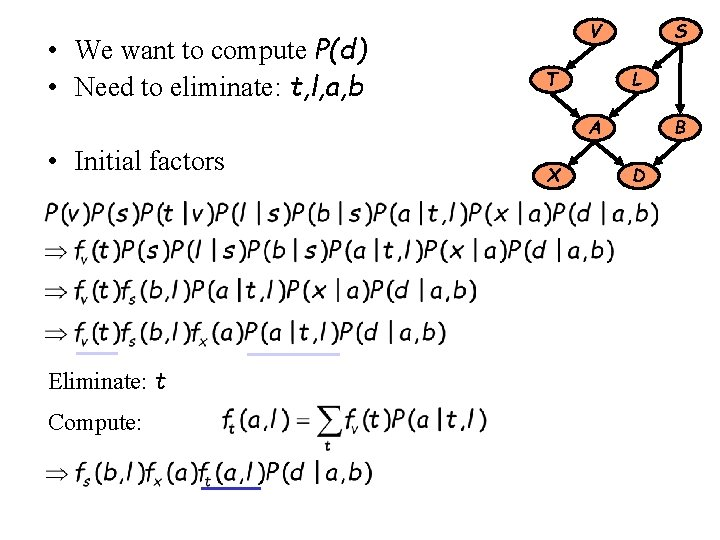• We want to compute P(d) • Need to eliminate: t, l, a, b S V L T B A • Initial factors Eliminate: t Compute: X D• We want to compute P(d) • Need to eliminate: l, a, b S V L T B A • Initial factors Eliminate: l Compute: X D• We want to compute P(d) • Need to eliminate: b S V L T B A • Initial factors Eliminate: a, b Compute: X DVariable Elimination • We now understand variable elimination as a sequence of rewriting operations • Actual computation is done in elimination step • Computation depends on order of elimination – Optimal elimination order can be computed—but is NP -hard to do so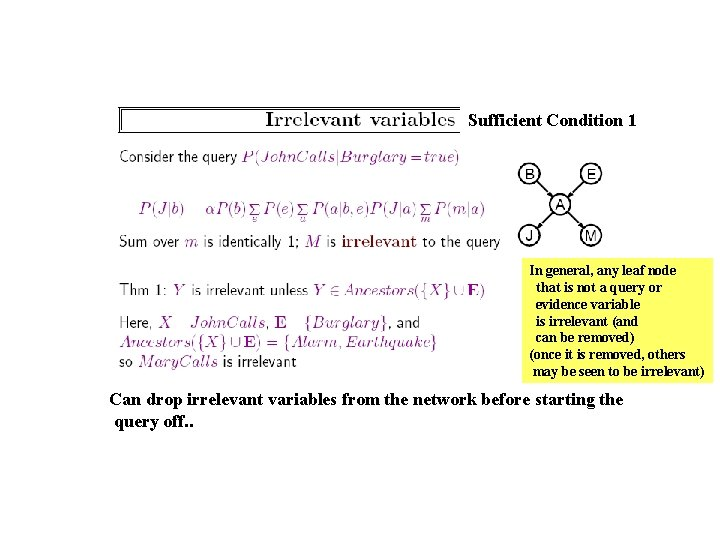Sufficient Condition 1 In general, any leaf node that is not a query or evidence variable is irrelevant (and can be removed) (once it is removed, others may be seen to be irrelevant) Can drop irrelevant variables from the network before starting the query off. .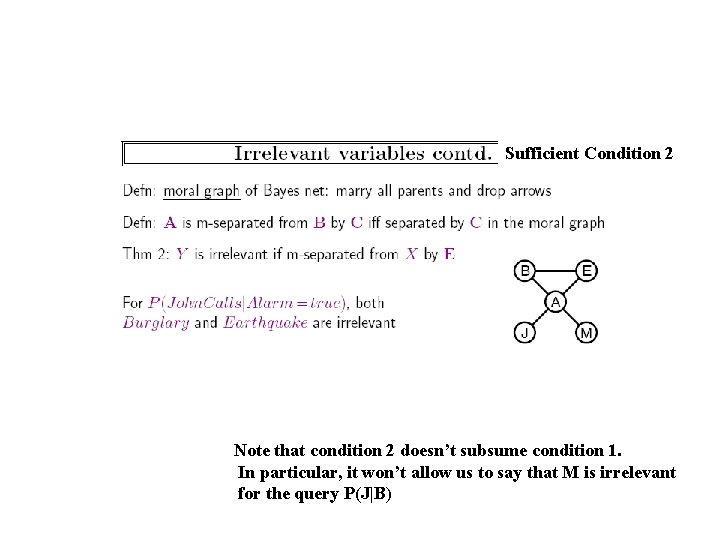Sufficient Condition 2 Note that condition 2 doesn’t subsume condition 1. In particular, it won’t allow us to say that M is irrelevant for the query P(J|B)